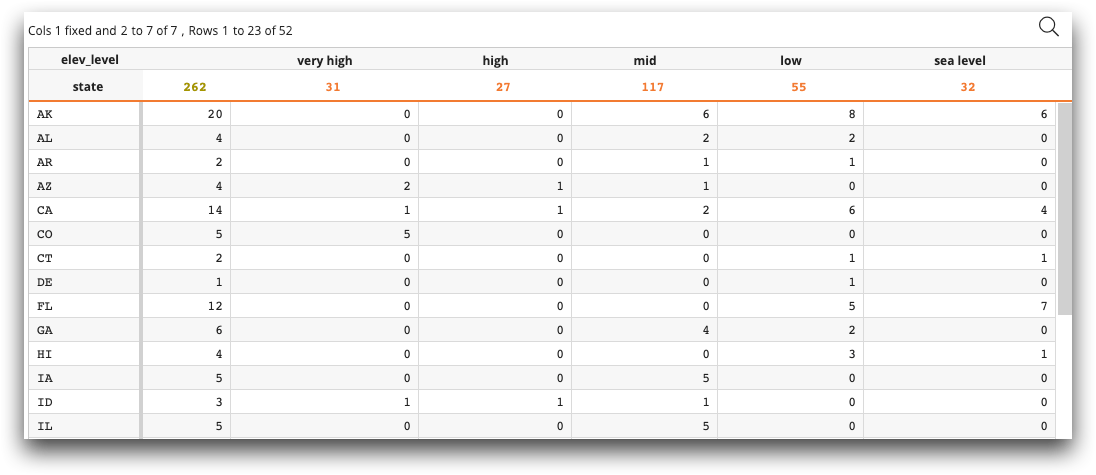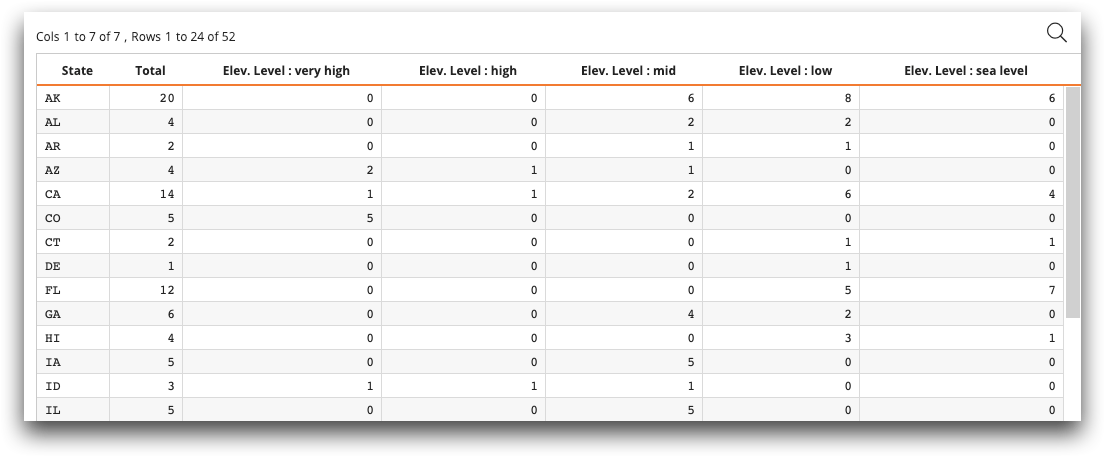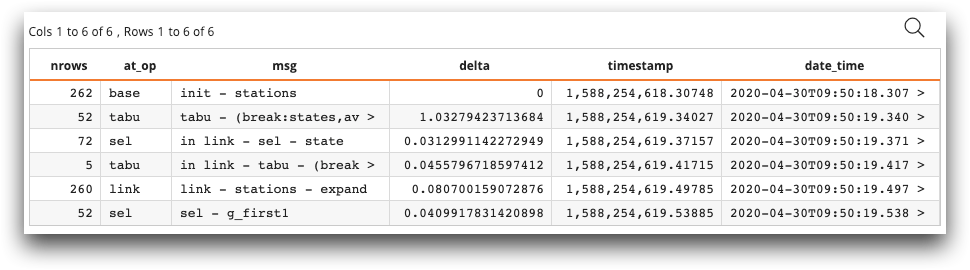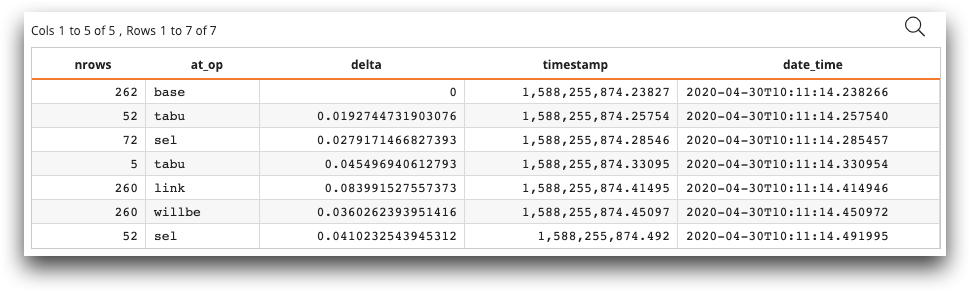# Macro Language examples

The following examples show how to implement various Python applications within Macro Language code with the functions `ten.GetData()`, `ten.MetaData()`, `ten.rows()`, and `ten.rebase()`.

This section contains various examples of Python applications within the ```<code language_=Python>``` tag of Macro Language. We start with a simple example that shows how to enter a Python session from Macro Language, perform modifications to a table in Python, and exit the Python session. The second example shows two different implementations of a logger operation.

## Example: A simple Python session

The following simple example demonstrates how to create a Macro Language query, enter a Python session, perform modifications to the table in Python, output a temporary table, and exit the Python session.

```<!--Perform a Macro Language query with a tabulation-->
<base table="demos.stations"/>
<willbe name="elev_level" value="range2list(elev;10,100,500,1000;'sea level','low','mid','high','very high')"/>
<tabu breaks="state" cbreaks="elev_level">
<tcol source="state" fun="cnt"/>
</tabu>

<!-- we enter the Python session here -->
<code language_="python">
<![CDATA[

# we create Metadata object md, GetData object gd, and DataFrame df
gd = ten.GetData()
df = gd.from_ops(ops).as_pandas()

# we set the metadata to ops (the state of the query at the <code> tag)
md.from_path(table,ops)

# we rename the column labels of the metadata
labs = md.labels[2:]
md.labels = 'State'
md.labels = 'Total'
md.labels[2:] = [s.replace('elev_level=','Elev. Level : ') for s in labs]
md.names[2:] = [s.replace('elev_level=','').replace(' ','_') for s in labs]

# we add the DataFrame and metadata to rebase to create a temporary table
ops = ten.rebase(df,md)
]]>

# we exit the Python session and continue with Macro Language
</code>```

In this example, we first create a query in Macro Language. We start with the base table `demos.stations`, create a column `elev_level`, and create a tabulation that breaks out each state's stations by elevation level (```sea level```, `low`, `mid`, `high`, and `very high`).

Before the Python session, the query looks like the following:We then invoke ```<code language_="python">```. We did not need to import any libraries, because `<code>` automatically imports `numpy`, `pandas`, `sklearn`, and `ten`. `ten` is a 1010data-supplied module that is loaded into the Python session.

As discussed in Python in 1010data: Basic syntax, the variables `ops` and `table` are loaded automatically into the Python session. `ops` in this case is the state of the table `demos.stations` before the `<code>` tag. `table` is simply `default.lonely`.

After the `<!CDATA[` tag, you can begin entering Python code. This Python code starts by instantiating the `MetaData` object `md` with `ten.MetaData()` and the `GetData` object `gd` with `ten.GetData()`. We use these objects to extract both metadata and raw data. We also create the pandas DataFrame `df` and assign it a value with the method `gd.from_ops(ops).as_pandas()`.

Next, we use the method `md.from_path(table,ops)` to set the metadata to the current state of the query.

Since we have a metadata object set to the current query, we can now change the metadata of the table. The next lines of code change the metadata `labels` for the columns of the table. The first two column labels are changed to `State` and `Total`. The rest of the column labels are changed to replace `elev_level=` with ```Elev. Level :```. The column names are also changed from the system-generated tabulation names (`m0`, `m1`, etc.) to more meaningful column names.

Finally, we can pass the resulting `DataFrame` and metadata to the `ten.rebase` method. The return value of `ten.rebase` is `ops`, which is a temporary table. The `rebase` method also returns back a list with a path and a dictionary. The path is our new worksheet and is in our temporary table cache. The dictionary has the same attributes as `<base>` would have.

The table after the Python session now looks like the following:Note that the temporary table contains the tabulation from the Macro Language code and the modified column labels and names from the Python code.

## Example: Building a logger

The following example shows how to build a logger operation with Python. Since the Python session is the same process as the 1010 process and the Python session doesn't close or end when the `<code>` tag is finished, we can treat our Python session as a cache. We can create an op in Python that caches some data with the various `GetData()` methods.

The Macro Language code uses `<defop>` to create two ops, `<initialize_logger>` and `<log>`. We create `<initialize_logger>` at the very beginning of the query, and scatter `<log>` throughout the query. See `<defop>` in the 1010data Reference Manual for more information.

```<base table="demos.stations"/>
<library>
<defop name="initialize_logger" msg="">
<code language_="python" msg="{@msg}">
<![CDATA[
import time
import ten
from datetime import datetime
class QueryLog():
def __init__(self,ops,table,message):
self.history = {k:[] for k in ['nrows','at_op','msg','delta','timestamp','date_time']}
self.history['nrows'].append(ten.rows(ops,table))
self.history['at_op'].append(ops[-1])
self.history['msg'].append(message)
t0 = time.time()
self.history['delta'].append(0)
self.history['timestamp'].append(t0)
self.history['date_time'].append(self.to_isoformat(t0))

@staticmethod
def to_isoformat(x): return datetime.fromtimestamp(x).isoformat()

def log_state(self,ops,table,message):
# note the order in which log_dic appends
self.history['nrows'].append(ten.rows(ops,table))
self.history['at_op'].append(ops[-1])
self.history['msg'].append(message)
t0 = time.time()
self.history['timestamp'].append(t0)
self.history['delta'].append(t0 - self.history['timestamp'][-2])
self.history['date_time'].append(self.to_isoformat(t0))

def get_log(self):
return self.history
# initializing our logger
q_log = QueryLog(ops, table, '~{@msg}~')
]]>
</code>
</defop>
<defop name="log" msg="">
<code language_="python" msg="{@msg}">
<![CDATA[
def tenten_eval_cover(ops,table,q_log):
q_log.log_state(ops,table,'~{@msg}~')
return (ops,table,q_log)

# The wrapper function tenten_eval_cover protects us from
# an optimized and sometimes unintuitive evaluation pattern
# that occurs in some ops (link, merge, etc...).
# To have total control of the scope of the evaluation you
# can use a wrapper function.
#
# Note: This example is educational, it would be cleaner
# to pass ops, and table as a return value from log_state().

ops,table,q_log = tenten_eval_cover(ops,table,q_log)
]]>
</code>
</defop>
</library>
<initialize_logger msg="init - stations"/>
<tabu breaks="state">
<tcol fun="avg" name="avg_elev" source="elev"/>
</tabu>
<log msg = " tabu - (break:states,avg elev) "/>
<sel value="state='CA''TX''NY''FL''AK'"/>
<log msg = " in link - sel - state" />
<tabu breaks="state">
<tcol fun="avg" name="avg_elev_big" source="elev"/>
</tabu>
<log msg = " in link - tabu - (break:states,avg elev)"/>
<log msg=" link - stations - expand "/>
<willbe name="similar_elev" value="abs(avg_elev_big-avg_elev)"/>
<sel value="g_first1(state;;similar_elev)"/>
<log msg=" sel - g_first1 "/>
<code language_="python">
<![CDATA[
#out to DataFrame
ops = ten.rebase(pd.DataFrame(q_log.get_log()))
]]>
</code>```

The resulting temporary table contains the log of the activity in the table `demos.stations`, including the number of rows affected by the operation, the type of operation, a user-defined log message, and the amount of time each operation took.## Example: Alternate logger

The following example also builds a logger, but makes use of `<entire_="1">` in the `<code>` tag. The `entire` flag drops the entire query in the `ops` value, not just the ops up to the current code tag, as was the case in the previous examples. By having the entire query in the ops, we can place our `<code>` tag for logging at the very beginning and take log measurements for many query slices.

Another advantage of using the `entire` flag is that once the `<code>` tag is finished, all results have been cached and we will not need to rerun the query. However, adding log messages and skipping ops is more difficult with this method.

```<base table="demos.stations"/>
<code language_="python" entire_="1">
<![CDATA[
# Using entire and recursion
#loop over slices of the query, where each slices starts at 0
# The name of ith op is stored in first index, ops[i]
# The dictionary of op attributes for the ith op is stored in the second index, ops[i]
#
import time
from datetime import datetime
def to_isoformat(x): return datetime.fromtimestamp(x).isoformat()
history = {k:[] for k in ['nrows','at_op','delta','timestamp','date_time']}
def log_query(ops,table,hist):
# determine the number of ops in the entire query in this scope!
nops = len(ops)
for i in range(0,nops):
if 'ops' in ops[i]:
log_query(ops[i]['ops'],ops[i]['table2'],hist)
history['nrows'].append(ten.rows(ops[0:i+1],table))
history['at_op'].append(ops[i])
t0 = time.time()
history['timestamp'].append(t0)
history['delta'].append(0 if len(history['delta']) == 0 else t0 - history['timestamp'][-2])
history['date_time'].append(to_isoformat(t0))

log_query(ops,table,history)
ops = ten.rebase(pd.DataFrame(history))
]]>
</code>
<tabu breaks="state">
<tcol fun="avg" name="avg_elev" source="elev"/>
</tabu>